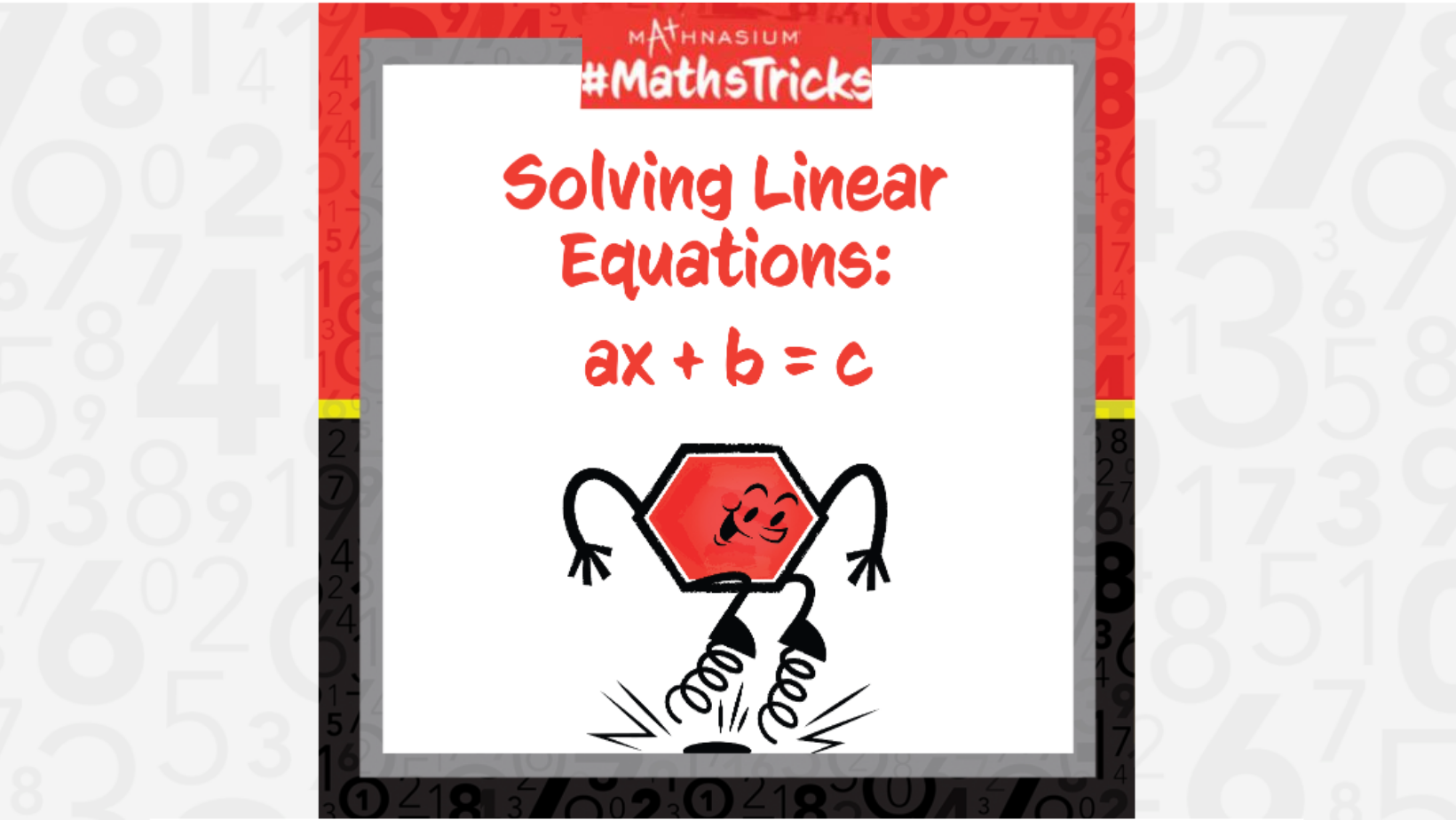0207 0787 247
Get Started by Finding a Local Centre

# Mathnasium #MathsTricks: Algebra (Solving Linear Equations Part 2)

Oct 1, 2023We can sometimes solve linear equations using inspection. When solving for x, we can think “something plus b equals c,” and “a times the number x equals that something.”

In some cases, it may be difficult to solve for x mentally. Instead, to solve for the variable x, we undo the operations in the reverse of the order of operations. That means we start by undoing the addition or subtraction first and then undoing the multiplication or division. Recall the Law of Equations tells us that operations done on one side of the equal sign must also be done on the other side of the equal sign to keep the equation in balance.

Follow the examples below to solve for the variable.

#### Example 1: -3n – 6 = 0.

Step 1: Undo the addition or subtraction on both sides of the equation.

-3n – 6 = 0

-3n – 6 + 6 = 0 + 6

-3n + 0 = 6

-3n = 6

Step 2: Undo the multiplication by dividing both sides of the equation by the coefficient.

-3n = 6

-3n/-3 = 6/-3

n = 6/-3

n = -2

Step 3: Check your work by plugging in for the value of the variable.

-3(-2) – 6 = ?

6 – 6 = 0

#### Example 2: 11y – 6 = -10.

Step 1: Undo the addition or subtraction on both sides of the equation.

11y – 6 = -10

11y – 6 + 6 = -10 + 6

11y + 0 = -4

11y = -4

Step 2: Undo the multiplication by dividing both sides of the equation by the coefficient.

11y = -4

11y/11 = -4/11

y = -4/11

Step 3: Check your work by plugging in for the value of the variable.

11(-4/11) – 6 = ?

-4 – 6 = -10

Now, with this strategy, you are ready to use this Mathnasium Maths Trick to solve linear equations in the form ax + b = c. Click here for more practise problems, then check your answers here.

If you missed this, or any of our other Maths Tricks videos, check them out on our YouTube channel!

Do you have a maths trick you want to see? Submit your request at: http://bit.ly/MathnasiumMathTricks.

## SEE HOW MATHNASIUM WORKS FOR YOUR SITUATION

### My Child is:## OUR METHOD WORKS

Mathnasium meets your child where they are and helps them with the customised program they need, for any level of mathematics.Early YearsPrimary SchoolSecondary SchoolGCSE